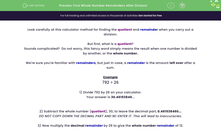# Find Whole Number Remainders After Division

In this worksheet, students state the whole number remainders after carrying out divisions.Key stage:  KS 3

Curriculum topic:   Number

Curriculum subtopic:   Use Calculators/Technology for Accuracy

Difficulty level:#### Worksheet Overview

Look carefully at this calculator method for finding the quotient and remainder when you carry out a division.

But first, what is a quotient?

Sounds complicated?  Do not worry, this fancy word simply means the result when one number is divided by another, or the whole number.

We're sure you're familiar with remainders, but just in case, a remainder is the amount left over after a sum.

Example

792 ÷ 26

1) Divide 792 by 26 on your calculator.

2) Subtract the whole number (quotient), 30, to leave the decimal part, 0.461538460...

DO NOT COPY DOWN THE DECIMAL PART AND RE-ENTER IT. This will lead to inaccuracies.

3) Now multiply the decimal remainder by 26 to give the whole number remainder of 12.

So 792 ÷ 26 = 30 remainder 12

(30 is the quotient and 12 is the remainder)

Let's give this a go!

### What is EdPlace?

We're your National Curriculum aligned online education content provider helping each child succeed in English, maths and science from year 1 to GCSE. With an EdPlace account you’ll be able to track and measure progress, helping each child achieve their best. We build confidence and attainment by personalising each child’s learning at a level that suits them.

Get started